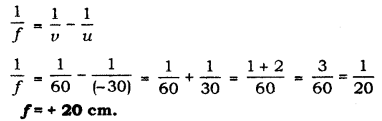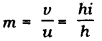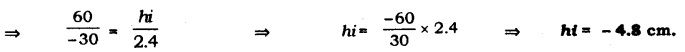# The image of a candle flame placed at a distance 30 cm from a spherical lens is formed on a screen

The image of a candle flame placed at a distance 30 cm from a spherical lens is formed on a screen placed at a distance of 60 cm from the lens. Identify the type of lens and calculate its focal length. If the height of the flame is 2.4 cm, find the height of its image

Here, u = - 30 cm, v = 60 cm, h = 2.4 cm
using lens formula,Positive focal length represents convex lens. Now,